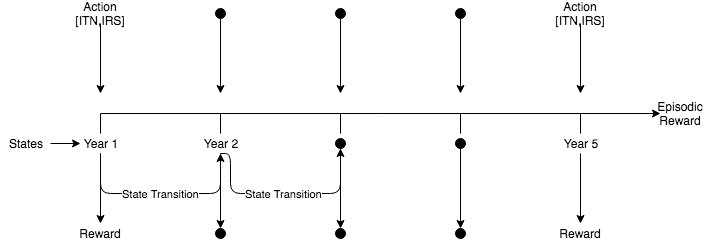## KDD Cup|Humanities Track Tutorial Q-Learning

Date Created: May 20, 2019

# KDD Cup|Humanities Track Tutorial Q-Learning

### State

$S \in \{1,2,3,4,5\}$

### Action

$A_S = [a_{\mathrm{ITN}}, a_{\mathrm{IRS}}]$ where $a_{\mathrm{ITN}} \in [0,1]$ and $a_{\mathrm{IRS}}\in [0,1]$

### Reward

$R_\pi \in (-\infty, \infty)$In [ ]:
import numpy as np
from collections import defaultdict
import random

from netsapi.challenge import *


# Learning a Value Function Based on $\epsilon$-greedy action selection¶

This common resource was used as a reference for the implementation presented here: https://kofzor.github.io/Learning_Value_Functions/. Please refer to the blog and this Tutorial in tandem. The code below uses the first example from the blog with the Challenge Environment (as opposed to Gym).

In [ ]:
env = ChallengeSeqDecEnvironment()

Q = defaultdict(lambda : 0.) # Q-function
n = defaultdict(lambda : 1.) # number of visits

def actionSpace(resolution):
x,y = np.meshgrid(np.arange(0,1+resolution,resolution), np.arange(0,1+resolution,resolution))
xy = np.concatenate((x.reshape(-1,1), y.reshape(-1,1)), axis=1)
return xy.round(2).tolist()

#HyperParameters
epsilon = 0.1
gamma = 0.9
action_resolution = 0.2
episode_number = 3 #for submission this is fixed as 20

#Set-up
actions = actionSpace(action_resolution)
actionspace = range(len(actions)-1)
greedy_action = lambda s : max(actionspace, key=lambda a : Q[(s,a)])
max_q = lambda sp : max([Q[(sp,a)] for a in actionspace])

#Training of Q Table
for _ in range(episode_number):
env.reset()
nextstate = env.state
while True:
state = nextstate

# Epsilon-Greedy
if epsilon > random.random() :
action = random.choice(actionspace)
print('random_action',action)
else :
action = greedy_action(state)

env_action = actions[action] #convert to ITN/IRS
print('env_action', env_action)
nextstate, reward, done, _ = env.evaluateAction(env_action)

# Q-learning
if done :
Q[(state,action)] = Q[(state,action)] + 1./n[(state,action)] * ( reward - Q[(state,action)] )
break
else :
Q[(state,action)] = Q[(state,action)] + 1./n[(state,action)] * ( reward + gamma * max_q(nextstate) - Q[(state,action)] )

#Greedy Policy Learnt from Q Table
best_policy = {state: list(actions[greedy_action(state-1)]) for state in range(1,6)}
best_reward = env.evaluatePolicy(best_policy)
print(best_policy, best_reward)


## Creating a Valid Submission from Agent Code:

In [ ]:
class Q_Agent():

def __init__(self, environment):

#Hyperparameters
self.env = environment
self.epsilon = 0.1
self.gamma = 0.9
self.action_resolution = 0.2
self.Q = defaultdict(lambda : 0.) # Q-function
self.n = defaultdict(lambda : 1.) # number of visits
self.actions = actionSpace(self.action_resolution)
self.actionspace = range(len(self.actions)-1)

def actionSpace(self):
x,y = np.meshgrid(np.arange(0,1+self.action_resolution,self.action_resolution), np.arange(0,1+self.action_resolution,self.action_resolution))
xy = np.concatenate((x.reshape(-1,1), y.reshape(-1,1)), axis=1)
return xy.round(2).tolist()

def train(self):

Q = self.Q
n = self.n
actions = self.actions
actionspace = self.actionspace

greedy_action = lambda s : max(actionspace, key=lambda a : Q[(s,a)])
max_q = lambda sp : max([Q[(sp,a)] for a in actionspace])

for _ in range(20): #Do not change

self.env.reset()
nextstate = self.env.state

while True:
state = nextstate

# Epsilon-Greedy Action Selection
if epsilon > random.random() :
action = random.choice(actionspace)
else :
action = greedy_action(state)

env_action = actions[action]#convert to ITN/IRS
print('env_action', env_action)
nextstate, reward, done, _ = self.env.evaluateAction(env_action)

# Q-learning
if done :
Q[(state,action)] = Q[(state,action)] + 1./n[(state,action)] * ( reward - Q[(state,action)] )
break
else :
Q[(state,action)] = Q[(state,action)] + 1./n[(state,action)] * ( reward + gamma * max_q(nextstate) - Q[(state,action)] )

return Q

def generate(self):
best_policy = None
best_reward = -float('Inf')

Q_trained = self.train()
greedy_eval = lambda s : max(actionspace, key=lambda a : Q_trained[(s,a)])

best_policy = {state: list(actions[greedy_eval(state-1)]) for state in range(1,6)}
best_reward = self.env.evaluatePolicy(best_policy)

print(best_policy, best_reward)

return best_policy, best_reward


## Run the EvaluateChallengeSubmission Method with your Agent Class

In [ ]:
EvaluateChallengeSubmission(ChallengeSeqDecEnvironment, Q_Agent, "Q_submission.csv")


There is now the opportunity to explore other such similar RL approaches, hyperparameter tuning or different action selection strategies for this family of approaches to the problem!